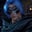Related Tags

julia

# How to get the complex conjugate of a complex number in JuliaGutha Vamsi Krishna

## Overview

A complex conjugate of a complex number in mathematics is a number that has the same real part (as that of the original complex number) and an imaginary part of equal magnitude (as that of the corresponding imaginary part of the original complex number) but with opposite signs.

If a+imb is a given complex number, then a-imb is its complex conjugate.

We can get the complex conjugate of a complex number in Julia using the conj() function.

### Syntax

conj(complex number)

### Parameter and return value

The conj() function takes a complex number as a parameter and returns its complex conjugate.

Let's take a look at an example of this.

### Example

#given complex number
z = 1 + 5im

#get complex conjugate
display(conj(z))
Complex conjugate in Julia

### Explanation

In the above code snippet:

• In line 2, we declare and initialize the complex number z.
• In line 5, we get the complex conjugate of the given complex number z using the function conj() and display the returned result.

RELATED TAGS

julia

CONTRIBUTORGutha Vamsi Krishna
RELATED COURSES

View all Courses

Keep Exploring

Learn in-demand tech skills in half the time